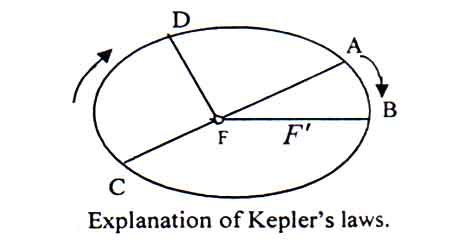# Explain Kepler’s law of planetary motion

A German astronomer, Johann Kepler, first stated the laws of planetary motion.

The three laws of planetary motion are:

1. The law of orbits: Every planet moves in an elliptical orbit having the sun at one of the foci.
2. The law of areas: The line joining any planet to the sun sweeps out equal areas in equal intervals of time. That is, the areal velocity of the planet is constant.
3. The law of periods: The square of the time period of revolution of a planet around the sun is proportional to the cube of the planet’s mean distance from the sun.

Explanation1st law: ABCD is an elliptical orbit. F and F’ are the two foci. According to the 1st law, the orbit of the planet is elliptical with the sun, as it’s one of the foci.

2nd law: Let the sun is at the focus F. If a planet takes time t coming from A to B, then it will take the same time to come from C to D.

Therefore, according to 2nd law,

Area of AFB = area of CFD

3rd law: Since the planets orbiting the sun in an elliptical orbit, so the distance of a planet will be different for different time.

Let the average distance of a planet from the sun = R and the planet take T time to round the sun once.

According to the 3rd Law, T ∝ R3